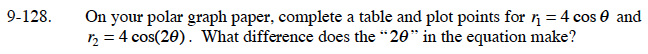### Home > CALC > Chapter 9 > Lesson 9.4.2 > Problem9-128

9-128.

On your polar graph paper, complete a table and plot points for r1 = 4 cosθ and r2 = 4 cos(2θ) . What difference does the "2θ " in the equation make? Homework Help ✎